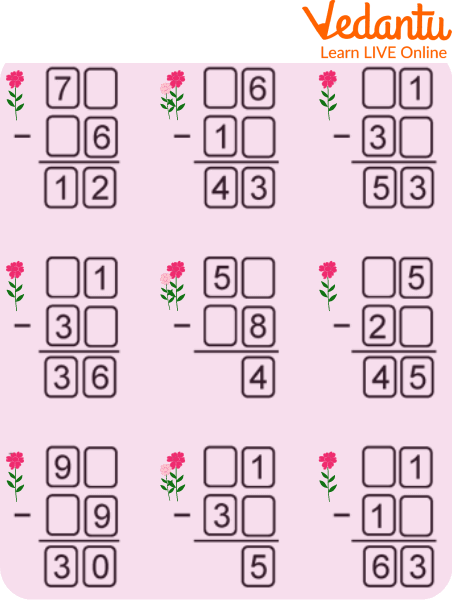Courses
Courses for Kids
Free study material
Offline Centres
More

# Missing Digit SubtractionLast updated date: 03rd Dec 2023
Total views: 102.9k
Views today: 1.02k## A Brief Note on Missing Digit Subtraction

The Missing Digit Subtraction is an extended version of normal subtraction. It performs all the applications of normal subtraction along with some more. This article contains all the information regarding missing digit subtraction. Some worksheets are also given for practice so that students get hold of the concepts tightly. The use of graphics and examples has made this learning interesting and easy. Let us now begin with the reading of this interesting topic.

## What is Missing Digit Subtraction?

Missing Digit Subtraction, like subtraction, is a statement involving an arithmetic operation, '-'. Missing digit subtraction consists of some missing digits, including the subtraction operation. Using the missing digit subtraction, one can find minuend, subtrahend, and difference. While in normal subtraction, no digit is missing, and it is used only to calculate the difference between two numbers.Missing digit subtraction

## Various rules to be followed to find the missing digit subtraction are given below:

1. If the missing digit is present just after the equality sign, then the difference is calculated by subtracting the subtrahend from the minuend

1. If the missing digit is present just before the equality sign, then the subtrahend is calculated by subtracting the difference from the minuend

1. If the missing digit is present at the starting point, before the equality sign, then the minuend is calculated by adding the subtrahend and the difference

## Solved Examples

Q 1. Calculate the missing term, 65 - __ 5 = 30.

Ans: To find the missing digit in the given problem, students must follow the below-given steps:

• Write the numbers, i.e. 65 - __ 5 = 30

• As the missing digit is present just before the equality sign, the subtraction of the difference from the minuend takes place

• Subtract the difference, i.e. 30, from the minuend, i.e. 65 to obtain the subtrahend, i.e. 65 - 35 = 30

• Now, compare the calculated subtrahend with the subtrahend whose digits are missing to obtain those missing digits, i.e. 35 and __ 5

Thus, the value of the missing digit is 3.Missing digit subtraction

Q 2. Find the missing digit of 2 __ - 12 = 15.

Ans: To solve the given problem, students must follow the below-given steps:

• Write the numbers, i.e. 2 __ - 12 = 15

• As the missing digit is at the starting point, so the addition of the subtrahend and the difference takes place

• Add up the subtrahend, i.e. 12 and the difference to obtain the minuend, i.e. 15 + 12 = 27

• Now, compare the calculated minuend with the minuend whose some digits are missing to obtain those missing digits, i.e. 27 and 2 __

Thus, the value of the missing digit is 7.

Q 3. Find the missing digit of 4 _ - _ 2 = 26.

Ans: First, we will write the numbers, i.e. 4 _ - _ 2 = 26.

• As the missing digit is once placed in the first row, and tens are placed in the second row. So now we will check what needs to be subtracted to get 6.

• So it will be 8. As 8 - 2 = 6.

• Similarly, now we will check what needs to be subtracted from 4 to get 2.

• So if 4 - 2 will be done. We will get 2 as a result.

• Therefore, 48 - 22 = 26.Missing 2 digit subtraction

## Missing Digit Subtraction Worksheets

Some Missing Digit Subtraction Worksheets are given below:Missing Digit Subtraction Worksheets

## Practice Problems

Numerous practice problems are assigned that need to be solved by the students themselves to clarify the concepts deeply.

Q 1: 8 - _____ = 4

Ans: 4

Q 2: 18 - _____ = 0

Ans: 18

Q 3: 27 - 7 = _____

Ans: 20

Q 4: _____ - 38 = 10

Ans: 48

Q 5: 51 - 30 = _____

Ans: 21

Q 6: _____ - 30 = 5

Ans: 35

## Summary

Subtraction is one of the most basic, widely used, and important mathematical operations. It helps in doing the calculations steadily. It is used to solve numerous word problems, determine the amount of substance left, etc. It has prime importance in one's daily life. It assists children in expanding their mental abilities and problem-solving skills. This article has covered all the topics related to missing digit subtraction and how to find the missing digit subtraction. Feel free to put your problems in the comment section.

## FAQs on Missing Digit Subtraction

1. What is subtraction?

Subtraction is a mathematical or arithmetic operation used to calculate the difference between two given numbers. The process of subtraction begins on the right side of a number and moves to the left side.  The symbol used to denote the difference between two numbers is '-'.

2. Is it possible to subtract two numbers having different signs?

Yes, it is quite possible to subtract two numbers with different signs because there is no relation between the subtraction of two numbers and their corresponding signs. It is easy to find the difference between two different sign numbers. For example, 3 - (-5) = 3 + 5 = 8.